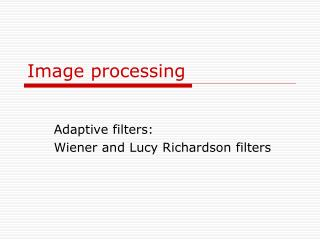DownloadDownload PresentationImage processing

# Image processing

Download Presentation## Image processing

- - - - - - - - - - - - - - - - - - - - - - - - - - - E N D - - - - - - - - - - - - - - - - - - - - - - - - - - -
##### Presentation Transcript

1. Image processing Adaptive filters: Wiener and Lucy Richardson filters

2. Image model • Original data = Image * PSF of blurring +additive noise • * - convolution operator The Importance of the PSF • Based on this model, the fundamental task of deblurring is to deconvolve the blurred image with the PSF that exactly describes the distortion. • The quality of the deblurred image is mainly determined by knowledge of the PSF.

3. Create a PSF of the blur and blur I = imread('flowers.tif'); figure; imshow(I); title('Original Image'); LEN = 31; % length of blur THETA = 11; % angle of blur PSF = fspecial('motion',LEN,THETA); %create PSF Blurred = imfilter(I,PSF,'circular','conv'); %blur figure; imshow(Blurred);title('Blurred Image'); imshow(imabsdiff(I,Blurred); % difference

4. Wiener filter • It removes the additive noise and inverts the blurring simultaneously. • The Wiener filtering is optimal in terms of the mean square error.

5. Filtering in the Frequency Domain 1. Transform image data to the frequency domain via the FFT 2. Multiply the image's spectrum with some filtering mask 3. Transform the spectrum back to the spatial domain that corresponds to deconvolution in the time domain

6. Wiener filter • Where Sxx (f1,f2), Sηη(f1,f2) are respectively power spectra of the original image and the additive noise, and H(f1,f2) is the blurring filter. • Wiener filter has two separate parts, an inverse filtering part and a noise smoothing part. • It performs the deconvolution by inverse filtering (highpass filtering) and removes the noise with a compression operation (lowpass filtering).

7. Power Spectrum • The most common way of generating a power spectrum is by using a Fourier transform and taking the square of the magnitude of the complex coefficients. • Other techniques such as the maximum entropy method can also be used to estimate the power spectrum.

8. Implementation • To implement the Wiener filter in practice we have to estimate the power spectra of the original image and the additive noise. • For white additive noise the power spectrum is equal to the variance of the noise. • To estimate the power spectrum of the original image many methods can be used. A direct estimate is the estimate of the power spectrum computed from the observation: • Where Y(k,l) is the DFT of the observation.

9. Discrete Fourier Transform

10. deblur the image by deconvolving wnr1 = deconvwnr(Blurred,PSF); figure;imshow(wnr1); title('Restored, True PSF');

11. Another blurring

12. Lucy-Richardson • Lucy-Richardson denoising accounts on photon counting noise with a Poisson distribution • There is also as you know the readout noise of Gaussian distribution

13. Lucy-Richardson • The Lucy-Richardson algorithm generates a restored image through an iterative method. • The essence of the iteration is as follows: the (n+1)th estimate of the restored image is given by the nth estimate of the restored image multiplied by a correction image. That is, original data image = image --------------- ● PSF* n+1 n image * PSF n

14. Implementation luc1 = deconvlucy(Blurred,PSF,5); figure; imshow(luc1); title('Restored Image, NUMIT = 5'); • deconvlucy function, by default, performs multiple iterations of the deblurring process. You can stop the processing, after a certain number of iterations, to check the result, and then restart the iterations from the point where processing stopped. The output cell array contains these four elements: output{1} -- The original input image output{2} -- The image produced by the last iteration output{3} -- The image produced by the next-to-last iteration output{4} -- Internal information used by deconvlucy to know where to restart the process

15. Result of 5 iterations

16. Result of 10 iterations

17. Image difference • imabsdiff